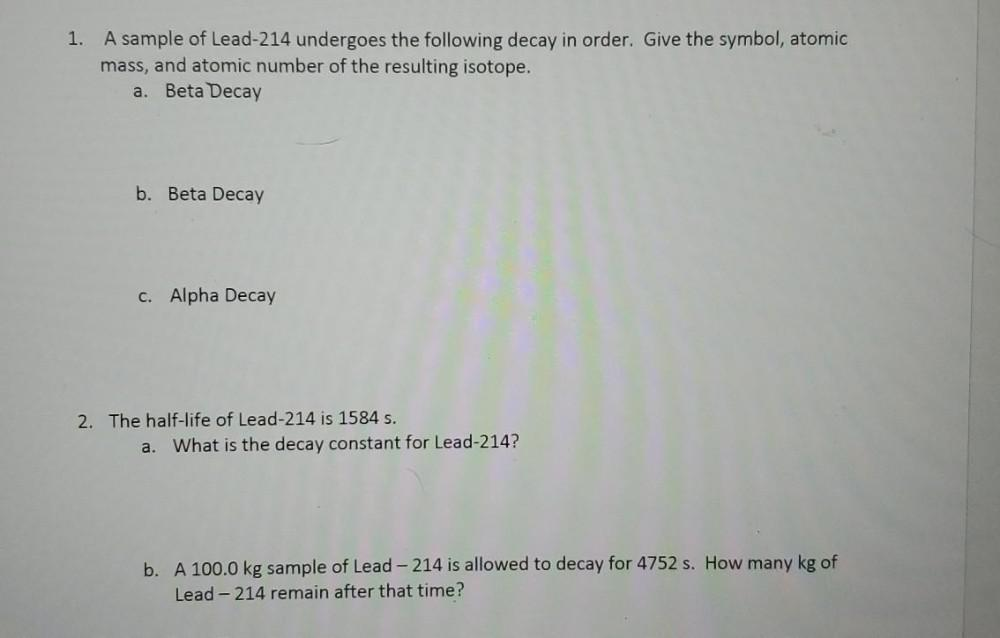Question:

# (1) A sample of Lead-214 undergoes the following decay in order. Give the symbol, atomic mass, and atomic number of the resulting isotope. (A) Beta Decay (B) Beta Decay (C) Alpha Decay (2) The half-li(1) A sample of Lead-214 undergoes the following decay in order. Give the symbol, atomic mass, and atomic number of the resulting isotope. (A) Beta Decay (B) Beta Decay (C) Alpha Decay (2) The half-life of Lead-214 is 1584 s. (A) What is the decay constant for Lead-214? (B) A 100.0 kg sample of Lead-214 is allowed to decay for 4752 s. How many kg of Lead-214 remain after that time?